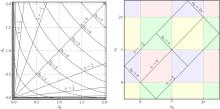# Rochester Institute of Technology

Site-wide linksNew Coordinates for the Amplitude Parameter Space of Continuous Gravitational Waves
By John T. Whelan Reinhard Prix Curt J. Cutler Joshua L. Willis
Published in Classical and Quantum Gravity 31, 065002 (Sunday, February 23, 2014)

## Abstract

The parameter space for continuous gravitational waves (GWs) can be divided into amplitude parameters (signal amplitude, inclination and polarization angles describing the orientation of the source, and an initial phase) and phase-evolution parameters (signal frequency and frequency derivatives, and parameters such as sky position which determine the Doppler modulation of the signal). The division is useful in part because of the existence of a set of functions known as the Jaranowski–Królak–Schutz (JKS) coordinates, which are a set of four coordinates on the amplitude parameter space such that the GW signal can be written as a linear combination of four template waveforms (which depend on the phase-evolution parameters) with the JKS coordinates as coefficients. We define a new set of coordinates on the amplitude parameter space, with the same properties, which can be more closely connected to the physical amplitude parameters. These naturally divide into two pairs of Cartesian-like coordinates on two-dimensional subspaces, one corresponding to left- and the other to right-circular polarization. We thus refer to these as circular polarization factored (CPF) coordinates. The corresponding two sets of polar coordinates (known as CPF-polar) can be related in a simple way to the physical parameters. A further coordinate transformation can be made, within each subspace, between CPF and so-called root-radius coordinates, whose radial coordinate is the fourth root of the radial coordinate in CPF-polar coordinates. We illustrate some simplifying applications for these various coordinate systems, such as a calculation of the Jacobian for the transformation between JKS or CPF coordinates and the physical amplitude parameters (amplitude, inclination, polarization and initial phase); a demonstration that the Jacobian between root-radius coordinates and the physical parameters is a constant; an illustration of the signal coordinate singularities associated with left- and right-circular polarization, which correspond to the origins of the two two-dimensional subspaces; and an elucidation of the form of the log-likelihood ratio between hypotheses of Gaussian noise with and without a continuous GW signal. These are used to illustrate some of the prospects for approximate evaluation of a Bayesian detection statistic defined by marginalization over the physical parameter space. Additionally, in the presence of simplifying assumptions about the observing geometry, we are able, using CPF-polar coordinates, to explicitly evaluate the integral for the Bayesian detection statistic, and compare it to the approximate results.

Whelan, John T.

## Research Areas

Gravitational Wave Astronomy